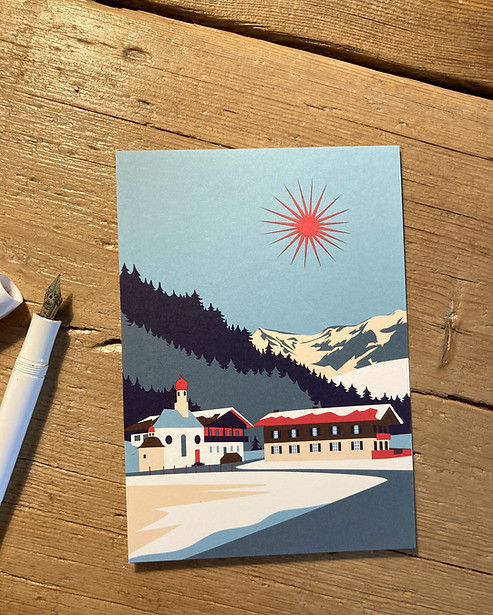top of pageW O L L D E C K E N

N E U

T E G E R N S E E

B U C H

F Ü R  K I N D E RT E G E R N S E E

P O S T K A R T E N## SCHAFKOPFKARTENB A D E H A N D T U C H

"T E G E R N S E E"S A G E N B U C HO B E R L A N D

P O S T K A R T E NM Ü N C H E N

P O S T K A R T E N

bottom of page